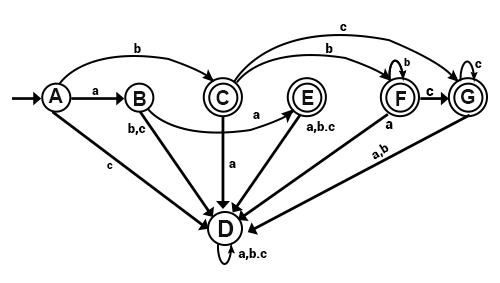# Construct NFA for the following language and convert it into DFA using the algorithm - L = (aa+ (bb*)c*)

Solution

NFA for the above language will be −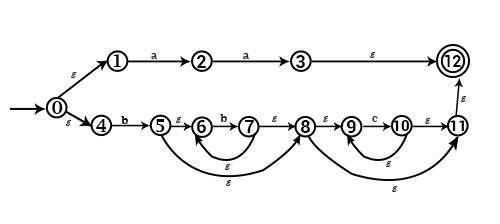Conversion from NFA to DFA −

ε − closure(0) = {0, 1, 4} = A

For State A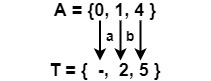For input symbol aFor input symbol bFor input symbol c
∴ Ta = {2}∴ Tb = {5}Tc = ∅
∴ ε − closure (Ta)
= ε
− closure (2)
= {2} = B
∴ ε − closure (Tb)
= ε
− closure (5)
= {5, 6, 8, 9, 11, 12} = C
∴ ε − closure (∅) = ∅
= D

For State B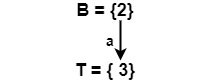For input symbol aFor input symbol bFor input symbol c
∴ Ta = {3}∴ Tb = {}Tc = {}
∴ ε − closure (3) =
= {3, 12} = E
∴ ε − closure (Tb) = { }
= ∅ = D
∴ ε − closure (Tc) = ∅ = D

For State C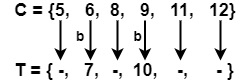For input symbol aFor input symbol bFor input symbol c
∴ Ta = {}∴ Tb = {7}Tc = {10}
∴ ε − closure (Ta) =
∅ = D
∴ ε − closure (7)
= { 7, 8, 6, 9, 11, 12}
= F
∴ ε − closure (10)
= {10, 9, 11, 12}
= G

For State E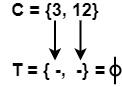∴ Ta = ∅ ∴ Tb = ∅ Tc = ∅ ∴ ε − closure (Ta) =             ∅ = D ∴ ε − closure (Tb) =                ф = D ∴ ε − closure (Tc) =               ф = D

For State F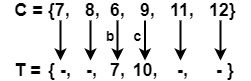∴ Ta = ∅ ∴ Tb = {7} Tc = {10} ∴ ε − closure (Ta) =        ∅ = D ∴ ε − closure (Tb) = = ε − closure (7)= {7,8,6,9,12}= F ∴ ε − closure (10) =    {10, 9, 11, 12} = G

For State G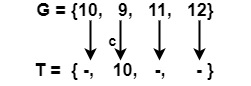∴ Ta = ∅ ∴ Tb = {7} Tc = {10} ∴ ε − closure (ф) = ф= D ∴ ε − closure (ф) = ф = D ∴ ε − closure (10) = G

For State D

D = ∅

Ta = Tb = Tc = ∅

ε − closure (Ta) = ε − closure (Tb) = ε − closure (Tc) = ф = D

Transition Table and Diagram for DFA will be −

(Initial State)

 a B c Here A B C D A = {0,1,4} B E D D B = {2} C D F G C = {5,6,8,9,11,12} D D D D D = ∅ E D D D E = {3, 12} F D F G F = {7,8,6,9,11,12} G D D G G = {10,9,11,12}